The goal of {tidyterra} is to provide common methods of the tidyverse packages for objects created with the {terra} package: SpatRaster and SpatVector. It also provides geoms for plotting these objects with {ggplot2}.

## Overview

{tidyverse} methods implemented on {tidyterra} works differently depending on the type of Spat* object:

• SpatVector: the methods are implemented taking advantage of the tidyverse implementation on {sf}. The SpatVector object is converted first to sf via sf::st_as_sf(), then the method (or function) is applied and finally the object is converted back to SpatVector with terra::vect(). Hence, rows correspond to geometries and columns correspond to attributes of the geometry.

• SpatRaster: The implementation on SpatRaster objects differs, since the methods could be applied to layers or to cells. {tidyterra} overall approach is to treat the layers as columns of a tibble and the cells as rows (i.e. select(SpatRaster, 1) would select the first layer of a SpatRaster).

The methods implemented return the same type of object used as input, unless the expected behavior of the method is to return another type of object, (for example, as_tibble() would return a tibble).

Current methods and functions provided by {tidyterra} are:

tidyverse method SpatVector SpatRaster
tibble::as_tibble() ✔️ ✔️
dplyr::select() ✔️ ✔️ Select layers
dplyr::mutate() ✔️ ✔️ Create /modify layers
dplyr::transmute() ✔️ ✔️
dplyr::filter() ✔️ ✔️ Modify cells values and (additionally) remove outer cells.
dplyr::slice() ✔️ ✔️ Additional methods for slicing by row and column.
dplyr::pull() ✔️ ✔️
dplyr::rename() ✔️ ✔️
dplyr::relocate() ✔️ ✔️
tidyr::drop_na() ✔️ ✔️ Remove cell values with NA on any layer. Additionally, outer cells with NA are removed.
tidyr::replace_na() ✔️ ✔️
ggplot2::geom_*() ✔️ geom_spatvector() ✔️ geom_spatraster() and geom_spatraster_rgb().

## ❗ A note on performance

{tidyterra} is conceived as a user-friendly wrapper of {terra} using the {tidyverse} methods and verbs. This approach therefore has a cost in terms of performance.

If you are a heavy user of {terra} or you need to work with big raster files, {terra} is much more focused on terms of performance. When possible, each function of {tidyterra} references to its equivalent on {terra}.

As a rule of thumb if your raster has less than 10.000.000 data slots counting cells and layers (i.e. terra::ncell(your_rast)*terra::nlyr(your_rast) < 10e6) you are good to go with {tidyterra}.

When plotting rasters, resampling is performed automatically (as terra::plot() does, see the help page). You can adjust this with the maxcell parameter.

## Installation

Install {tidyterra} from CRAN:

install.packages("tidyterra")

You can install the development version of {tidyterra} like so:

remotes::install_github("dieghernan/tidyterra")

Alternatively, you can install {tidyterra} using the r-universe:

# Enable this universe
options(repos = c(
dieghernan = "https://dieghernan.r-universe.dev",
CRAN = "https://cloud.r-project.org"
))
install.packages("tidyterra")

## Example

This is a basic example which shows you how to manipulate and plot SpatRaster objects:

library(tidyterra)

library(terra)

# Temperatures
f <- system.file("extdata/cyl_temp.tif", package = "tidyterra")

rastertemp <- rast(f)

library(ggplot2)

# Facet all layers

ggplot() +
geom_spatraster(data = rastertemp) +
facet_wrap(~lyr, ncol = 2) +
scale_fill_whitebox_c(
palette = "muted",
labels = scales::label_number(suffix = "º")
) +
labs(fill = "Avg temperature")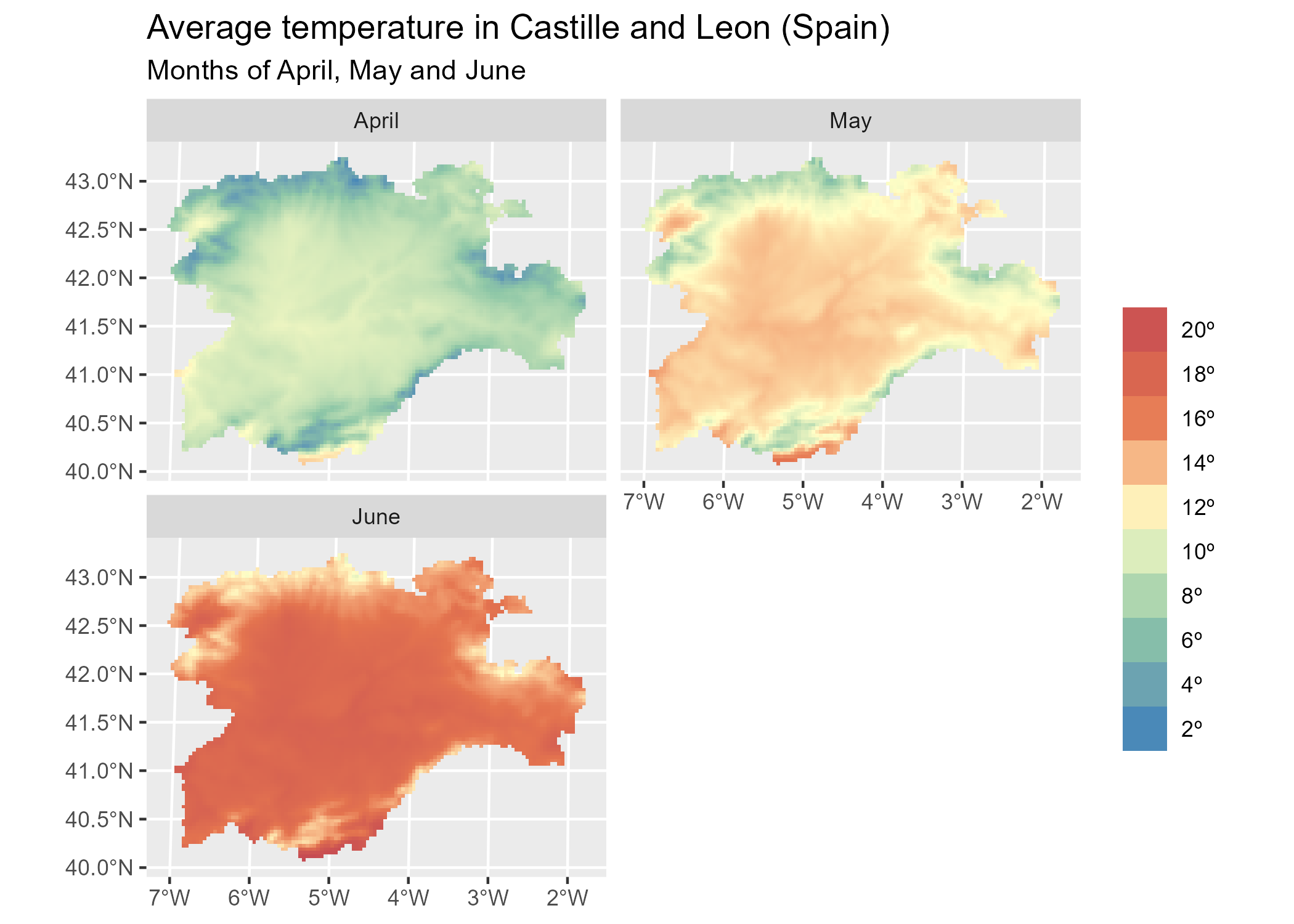# Create maximum differences

variation <- rastertemp %>%
mutate(
diff = tavg_06 - tavg_04
) %>%
select(var_apr_jun = diff)

# Add also a overlay of a SpatVector
f_vect <- system.file("extdata/cyl.gpkg", package = "tidyterra")

prov <- vect(f_vect)

ggplot() +
geom_spatraster(data = variation) +
geom_spatvector(data = prov, fill = NA) +
scale_fill_whitebox_c(
palette = "deep", direction = -1,
labels = scales::label_number(suffix = "º")
) +
theme_minimal() +
coord_sf(crs = 25830) +
labs(
fill = "Difference",
title = "Variation of temperature in Castille and Leon (Spain)",
subtitle = "(Average) temperatures in June vs. April"
)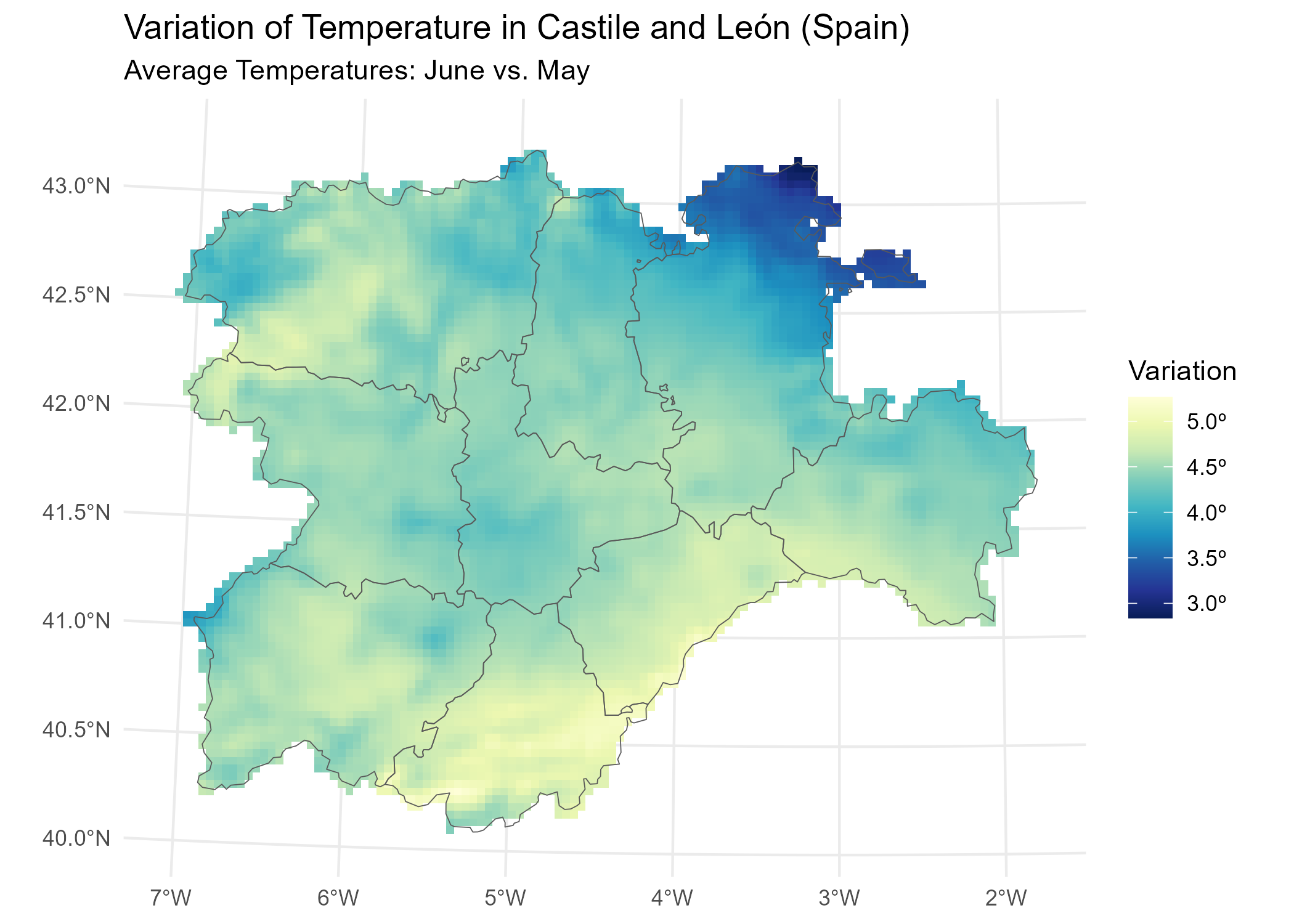{tidyterra} also provide a geom for plotting RGB SpatRaster tiles with {ggplot2}

f_tile <- system.file("extdata/cyl_tile.tif", package = "tidyterra")

rgb_tile <- rast(f_tile)

ggplot() +
geom_spatraster_rgb(data = rgb_tile) +
geom_spatvector(data = prov, fill = NA) +
theme_light()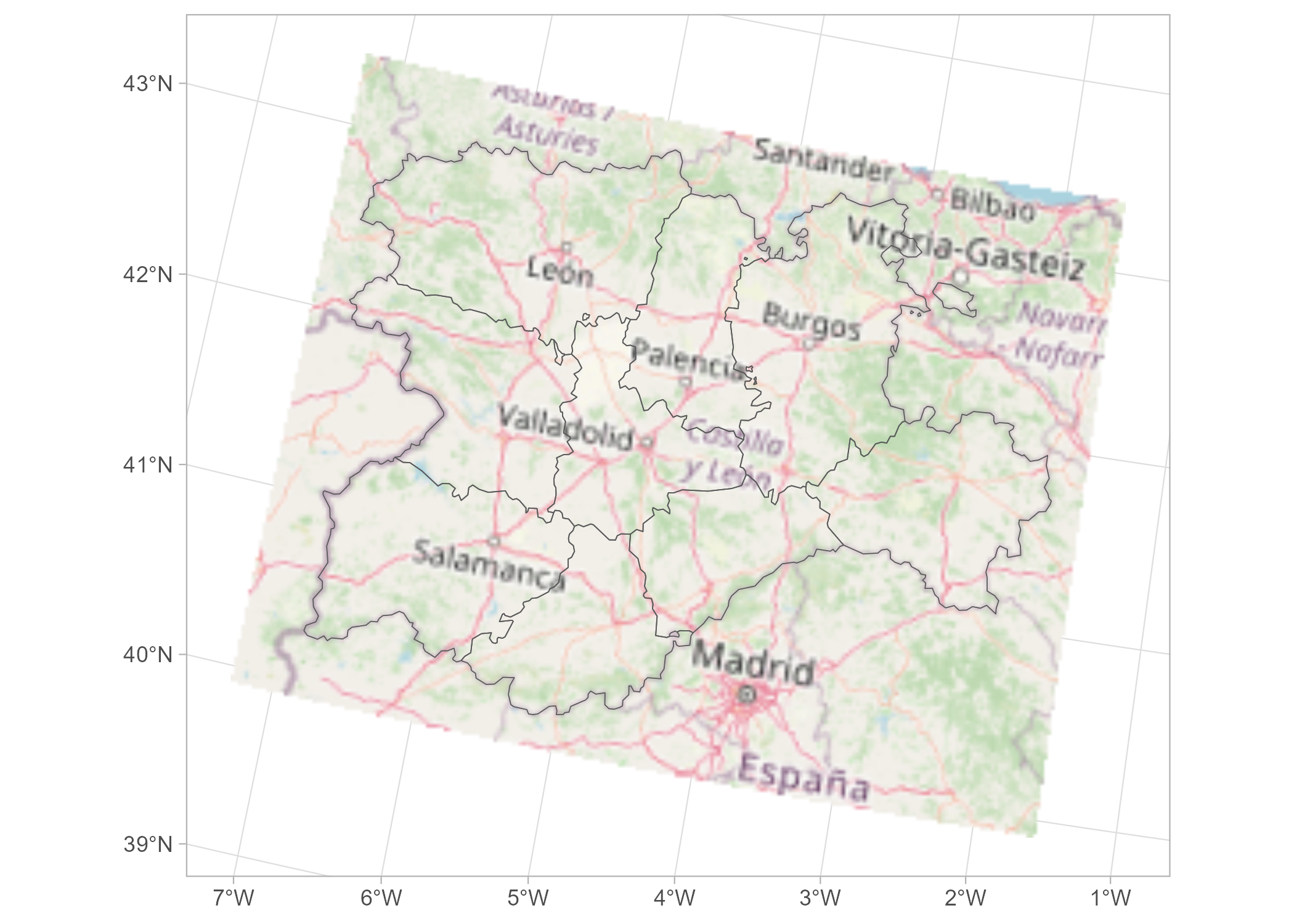# Recognizes coord_sf()

ggplot() +
geom_spatraster_rgb(data = rgb_tile) +
geom_spatvector(data = prov, fill = NA) +
theme_light() +
# Change crs and datum (for relabeling graticules)
coord_sf(crs = 3035, datum = 3035)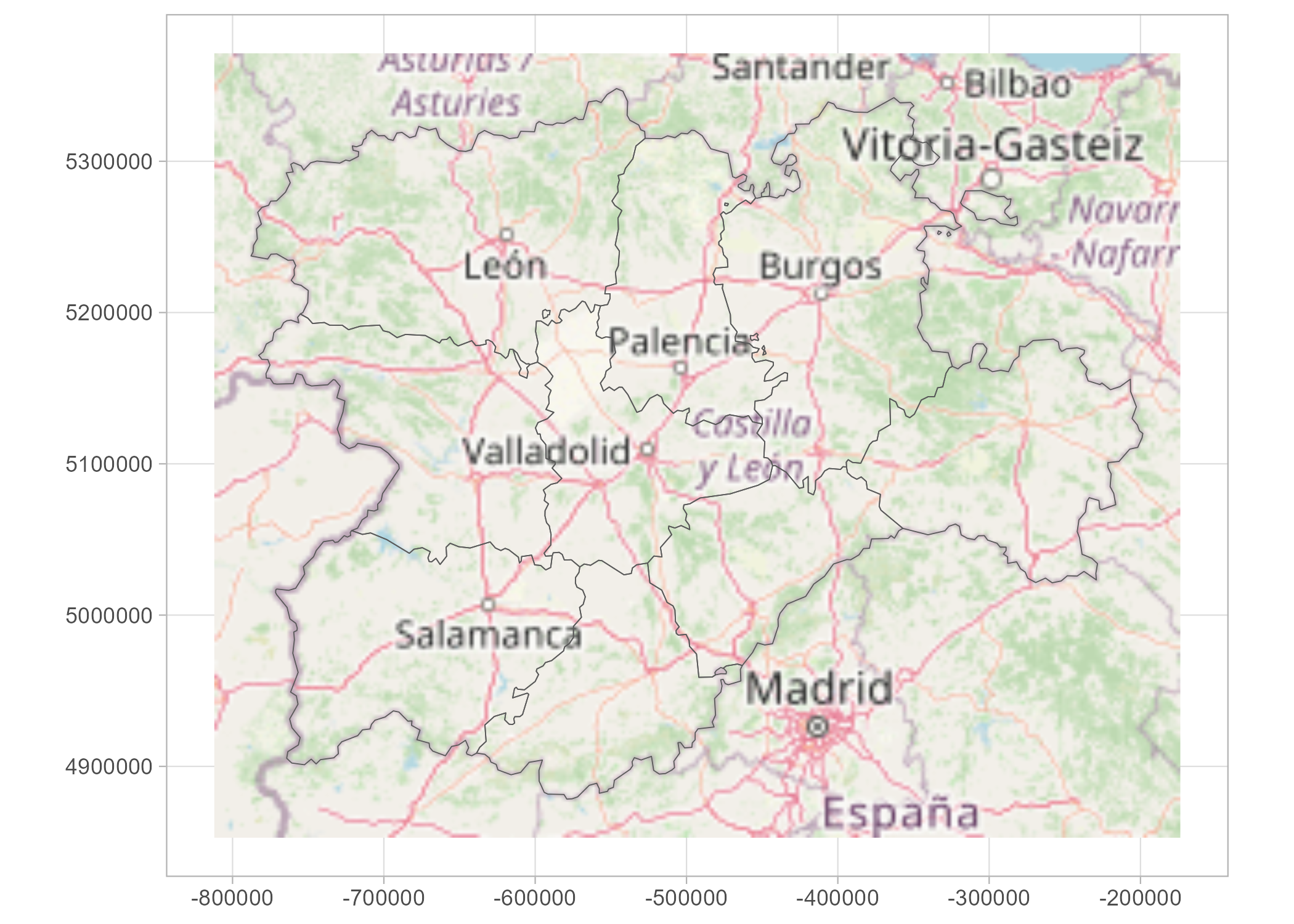{tidyterra} provides specific scales for plotting hypsometric maps with {ggplot2}:

asia <- rast(system.file("extdata/asia.tif", package = "tidyterra"))

terra::plot(asia)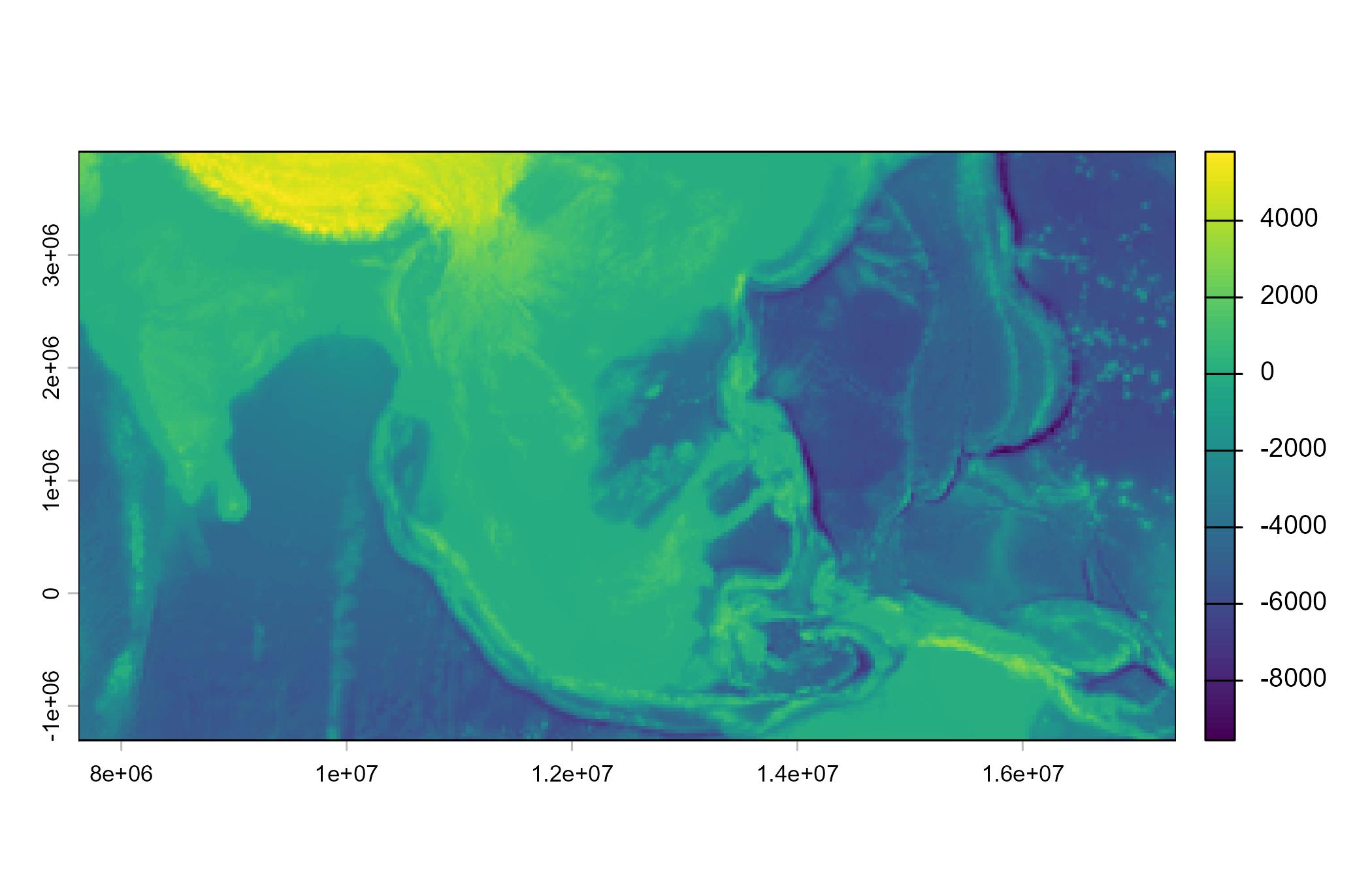ggplot() +
geom_spatraster(data = asia) +
scale_fill_hypso_tint_c(
palette = "gmt_globe",
labels = scales::label_number(),
breaks = c(-10000, -5000, 0, 2500, 5000, 8000),
guide = guide_colorbar(
direction = "horizontal",
title.position = "top",
barwidth = 20
)
) +
labs(
fill = "elevation (m)",
title = "Hypsometric map of Asia"
) +
theme_minimal() +
theme(legend.position = "bottom")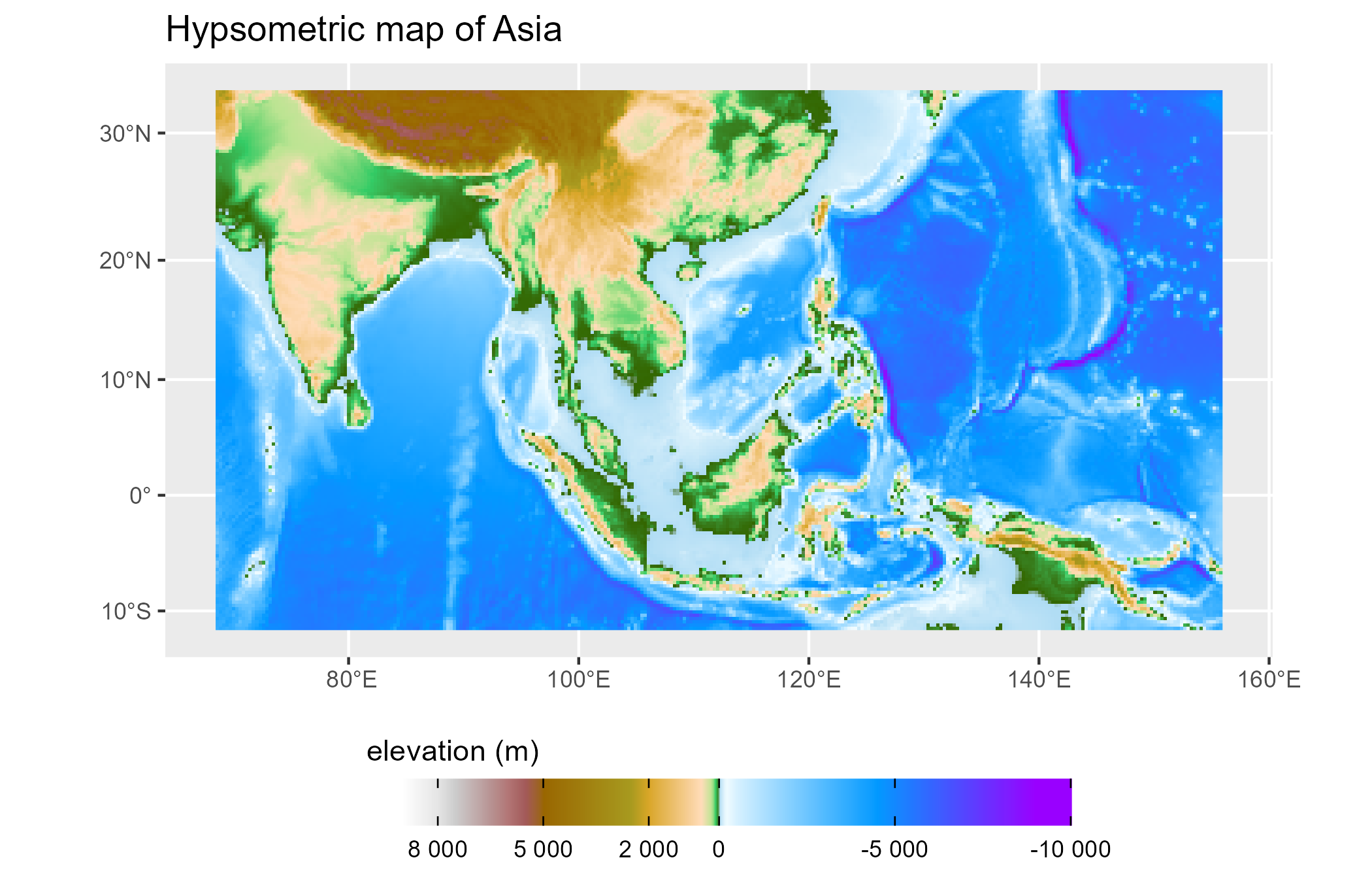{tidyterra} is currently on development mode. Please leave your feedback or open an issue on https://github.com/dieghernan/tidyterra/issues.

## Citation

To cite ‘tidyterra’ in publications use:

Hernangomez D (2022). tidyterra: tidyverse Methods and ggplot2 Utils for terra Objects. https://doi.org/10.5281/zenodo.6572471, https://dieghernan.github.io/tidyterra/

A BibTeX entry for LaTeX users is

@Manual{R-tidyterra,
title = {{tidyterra}: tidyverse Methods and ggplot2 Utils for terra Objects},
doi = {10.5281/zenodo.6572471},
author = {Diego Hernangómez},
year = {2022},
version = {0.2.0},
url = {https://dieghernan.github.io/tidyterra/},
abstract = {Extension of the tidyverse for SpatRaster and SpatVector objects of the terra package. It includes also new geom_ functions that provide a convenient way of visualizing terra objects with ggplot2.},
}

## Acknowledgements

{tidyterra} ggplot2 geoms are based on {ggspatial} implementation, by Dewey Dunnington and ggspatial contributors.